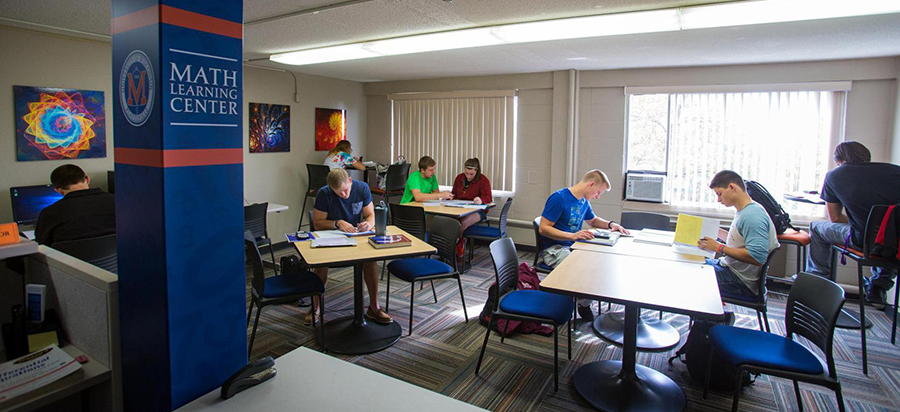The Mathematics Learning Center (MLC) at the University of Wisconsin-Platteville is a walk-in tutoring service located in 360 Gardner Hall. All tutors are capable math students in their third or fourth year of study. They can assist you with questions on homework and provide clarification on concepts. Tutoring is available for the following courses:

• MATH 1530 College Algebra
• MATH 1630 Finite Mathematics with Applications
• MATH 1730 Math of Finance*
• MATH 1830 Elementary Statistics*
• MATH 1930 Mathematical Explorations
• MATH 2450 Pre-calculus
• MATH 2530 Trigonometry and Analytic Geometry
• MATH 2630 Calculus with Applications
• MATH 2640 Calculus and Analytic Geometry I
• MATH 2740 Calculus and Analytic Geometry II
• MATH 2840 Calculus and Analytic Geometry III

*A tutor for elementary statistics is only available during select hours.

Additionally, tutoring is available for some upper level courses at select times. Check with the tutors for specifics.

If you are interested in working as a tutor in the MLC or ASC, you are encouraged to apply during the first week of each semester. Please contact deist@uwplatt.edu for more information.

Elementary Statistics and Math of Finance Tutors

We have tutors that specialize in Elementary Statistics and Math of Finance. These tutors are not available at all times, but you will find them in the Mathematics Learning Center.

Contact Information

Mathematics

0435 Gardner Hall